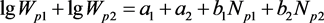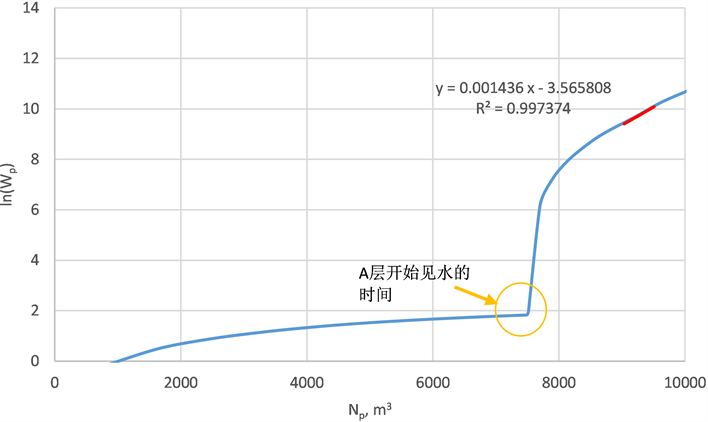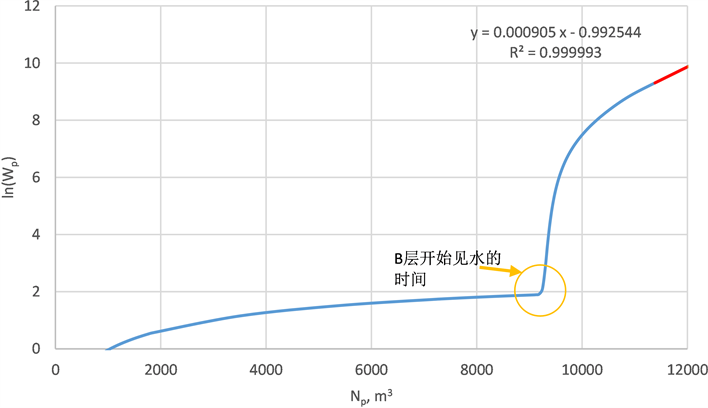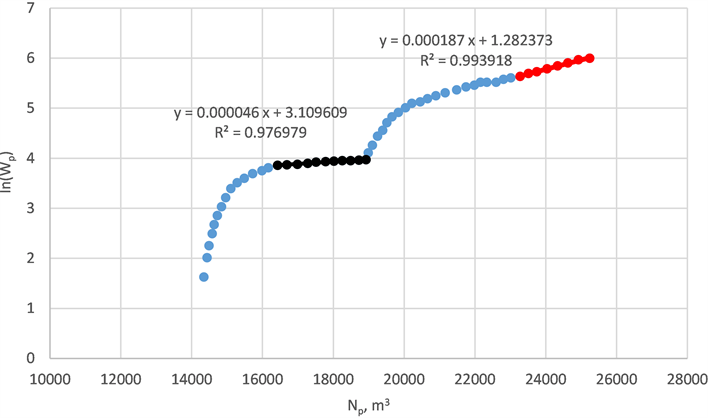水驱曲线在复杂多层油藏中的适应性分析Study of Water Flooding Curve Applied in Complicated Multi-Layers Reservoir

• 全文下载: PDF(574KB)    PP.722-729   DOI: 10.12677/AG.2018.84077
• 下载量: 218  浏览量: 344   科研立项经费支持

Water flooding curve is one of the most commonly used reservoir engineering method. Many re-searchers devote themselves to study theoretical basis, application conditions and methods to improve accuracy of water flooding curve. At present, different kinds of water flooding curves were proposed by many scholars, but few studies focused on adaptation of water flooding curve on complicated multi-layers reservoir. In the paper, theoretical basis of water flooding curve was discussed, then adaptation of water flooding curve on complicated multi-layers reservoir was analyzed in view of mathematics. On the basis of that, existing problems and solutions were given when water flooding curve was used in complicated multi-layer reservoir. Validity of the method proposed was verified by sensitivity analysis of numerical simulation and actual production data of some typical complicated multi-layer carbonate reservoir in the Middle East. The proposed method has reference significance for index forecast and dynamic analysis of complicated multi-layer reservoir.

1. 引言

2. 水驱曲线理论基础分析

$\frac{{K}_{ro}}{{K}_{rw}}=a{\left(\frac{1-{S}_{wi}}{1-{S}_{wi}-{S}_{or}}-{\stackrel{¯}{S}}_{wd}\right)}^{b}\text{\hspace{0.17em}}\text{\hspace{0.17em}}\text{\hspace{0.17em}}\left(b>0\right)$ (1)

 (2)

$\frac{{K}_{ro}}{{K}_{rw}}=a{\left({\stackrel{¯}{S}}_{wd}-b\right)}^{-\frac{1+n}{n}}\text{\hspace{0.17em}}\text{\hspace{0.17em}}\text{\hspace{0.17em}}\left(b>0,\text{\hspace{0.17em}}n>0\right)$ (3)

$\frac{{K}_{ro}}{{K}_{rw}}=\frac{a}{{\stackrel{¯}{S}}_{wd}}\mathrm{exp}{\left(-b{\stackrel{¯}{S}}_{wd}\right)}^{n}\text{\hspace{0.17em}}\text{\hspace{0.17em}}\text{\hspace{0.17em}}\left(b>0,\text{\hspace{0.17em}}n>0\right)$ (4)

$\frac{{K}_{ro}}{{K}_{rw}}=\frac{a}{1+b{\stackrel{¯}{S}}_{wd}}\mathrm{exp}\left(-b{\stackrel{¯}{S}}_{wd}\right)\text{\hspace{0.17em}}\text{\hspace{0.17em}}\text{\hspace{0.17em}}\left(b>0\right)$ (5)

${\stackrel{¯}{S}}_{w}=\frac{{N}_{p}}{N}\left(1-{S}_{wi}\right)+{S}_{wi}$ (6)

a) 水驱特征曲线公式1——纳札洛夫水驱曲线，推荐用于低粘(小于3 mPa∙s)层状油藏和碳酸盐岩底水驱油藏。其表达式为

 (7)

b) 水驱特征曲线公式2——马克西莫夫–童宪章水驱曲线，推荐用于中粘(3~30 mPa∙s)层状油藏。其表达式为

$\mathrm{lg}{W}_{p}=a+b{N}_{p}$ (8)

c) 水驱特征曲线公式3——西帕切夫水驱曲线，推荐用于中粘(3~30 mPa∙s)层状油藏。其表达式为

$\frac{{L}_{p}}{{N}_{p}}=a+b{L}_{p}$ (9)

d) 水驱特征曲线公式4——沙卓诺夫水驱曲线，推荐用于高粘(大于30 mPa∙s)层状油藏。其表达式为

$\mathrm{lg}{L}_{p}=a+b{N}_{p}$ (10)

e) 水驱特征曲线公式5——张金庆水驱曲线，适用于任何原油粘度和类型的水驱油藏。其表达式为

$\frac{{W}_{p}}{{N}_{p}}=-a+b\frac{{W}_{p}}{{N}_{p}^{2}}$ (11)

f) 水驱特征曲线公式6——俞启泰水驱曲线，适用于任何原油粘度和类型的水驱油藏。其表达式为

$\mathrm{lg}{N}_{p}=a-b\mathrm{lg}\left(\frac{{L}_{p}}{{W}_{p}}\right)$ (12)

1) 水驱曲线寻求的是动态指标的直线关系，以在应用时更加方便快捷；

2) 水驱曲线的理论基础是物质平衡方程和相渗曲线表达式；

3) 水驱曲线一般有一定的适用条件如油水粘度比等，同时需出现直线段，一般需到中高含水期。

3. 复杂多层油藏水驱曲线适应性分析

3.1. 双层油藏中常用的六类水驱曲线

$\frac{{L}_{p1}}{{N}_{p1}}={a}_{1}+{b}_{1}{W}_{p1}$$\frac{{L}_{p2}}{{N}_{p2}}={a}_{2}+{b}_{2}{W}_{p2}$ (13)

$\frac{{L}_{p1}}{{N}_{p1}}+\frac{{L}_{p2}}{{N}_{p2}}={a}_{1}+{a}_{2}+{b}_{1}{W}_{p1}+{b}_{2}{W}_{p2}$ (14)(15)

$\mathrm{lg}{L}_{p1}+\mathrm{lg}{L}_{p2}={a}_{1}+{a}_{2}+{b}_{1}{N}_{p1}+{b}_{2}{N}_{p2}$ (16)

$\frac{{L}_{p1}}{{N}_{p1}}+\frac{{L}_{p2}}{{N}_{p2}}={a}_{1}+{a}_{2}+{b}_{1}{L}_{p1}+{b}_{2}{L}_{p2}$ (17)

$\frac{{W}_{p1}}{{N}_{p1}}+\frac{{W}_{p2}}{{N}_{p2}}=-\left({a}_{1}+{a}_{2}\right)+{b}_{1}\frac{{W}_{p1}}{{N}_{p1}^{2}}+{b}_{2}\frac{{W}_{p2}}{{N}_{p2}^{2}}$ (18)

$\mathrm{lg}{N}_{p1}+\mathrm{lg}{N}_{p2}={a}_{1}+{a}_{2}-{b}_{1}\mathrm{lg}\left(\frac{{L}_{p1}}{{W}_{p1}}\right)-{b}_{2}\mathrm{lg}\left(\frac{{L}_{p2}}{{W}_{p2}}\right)$ (19)

3.2. 双层油藏水驱曲线可以叠加的特例

$\frac{{W}_{p1}}{{N}_{p1}}+\frac{{W}_{p2}}{{N}_{p2}}=-\left({a}_{1}+{a}_{2}\right)+{b}_{1}\frac{{W}_{p1}}{{N}_{p1}^{2}}+{b}_{2}\frac{{W}_{p2}}{{N}_{p2}^{2}}$ (20)

$\frac{{W}_{p2}}{{N}_{p2}}=-\left({a}_{1}+{a}_{2}\right)+{b}_{2}\frac{{W}_{p2}}{{N}_{p2}^{2}}$ (21)

4. 复杂多层油藏多层动态特征及数模验证

5. 复杂多层油藏水驱曲线预测实例分析Table 1. Basic parameters of mechanism simulation modelFigure 1. First type water flooding curve analysis of simulation results from mechanism modelFigure 2. First type water flooding curve of layer A after cumulative production splitFigure 3. First type water flooding curve of layer B after cumulative production split

B3和B4，各油组之间物性差异较大，详见图4，从图中可以看出，该油藏渗透率极差为35，纵向非均质性较强。Figure 4. Well log permeability in all oil zone of X oilfield in Middle EastFigure 5. First type water flooding curve of typical well with multi-layer commingle production of X oilfield in Middle East

6. 结论

1) 水驱曲线的理论基础是相渗曲线表达式及物质平衡方程。水驱曲线的系数隐含着不同的物性参数、不同的相渗参数以及不同的流体参数，本文探讨了其在纵向强非均质复杂多层油藏适用性，指出利用水驱曲线直接进行拟合预测误差较大；

2) 本文给出了复杂多层油藏水驱曲线的方法，其核心思想是分层、分阶段预测再叠加，通过数值模拟和敏感性方案分析，本文的提出方法可以一定程度上拓宽水驱曲线的实用性，对于复杂多层油藏指标预测具有一定的参考意义。

  陈元千. 水驱特征曲线关系式的推导[J]. 石油学报, 1985, 6(2): 69-78.  陈元千. 一种新型水驱特征曲线关系式的推导及应用[J]. 石油学报, 1993, 14(2): 65-73.  任玉林. 一种新的广义水驱曲线[J]. 新疆石油地质, 2006, 27(2): 188-190.  俞启泰. 几种重要水驱特征曲线的油水渗流特征[J]. 石油学报, 1999, 20(1): 56-60.  张金庆. 水驱油田产量预测模型[M]. 北京: 石油工业出版社, 2013: 19-48.  张金庆, 安桂荣, 许家峰, 刘晨. 广适水驱曲线适应性分析及推广应用[J]. 中国海上油气, 2013, 25(6): 56-60.  张金庆, 许家峰, 安桂荣, 甯波. 高含水油田适时产液结构优化调整计算方法[J]. 大庆石油地质与开发, 2013, 32(6): 76.  刘晨, 张金庆, 王凯, 周文胜. 张型广适与甲型水驱特征曲线关系研究[J]. 特种油气藏, 2017, 24(1): 106-109.  姜汉桥, 姚军, 姜瑞忠. 油藏工程原理与方法[M]. 东营: 中国石油大学出版社, 2006: 236-245.  赵斌, 王斌, 曾丽, 杨志远, 韩朝阳, 马晓燕. 利用水驱特征曲线确定剩余油分布研究[J]. 石油地质与工程, 2010, 24(3): 59-61.  周凤军, 葛丽珍, 王刚, 童凯军. 基于水驱特征曲线计算油水相对渗透率曲线的新方法[J]. 复杂油气藏, 2017, 10(1): 50-54.  高文君, 刘瑛. 产量递减规律与水驱特征曲线的关系[J]. 断块油气田, 2002, 9(3): 45-49.  李正民, 孙来喜, 邓虎城, 张娟, 李银婷. 水驱特征曲线的适用条件研究[J]. 物探化探计算技术, 2012, 34(2): 143-146.  孙玉凯, 高文军. 著常用油藏工程方法改进与应用[M]. 北京: 石油工业出版社, 2007: 58-79.  俞启泰. 一种广义水驱特征曲线[J]. 石油勘探与开发, 1998, 25(5): 48-50.